# Locally Weighted Regression

## Introduction

When studying regression algorithms in machine learning, we only touch over a few regression algorithms, but we miss out on a fundamental regression algorithm, i.e., Locally Weighted Regression. It is a non-parametric algorithm, unlike a typical linear regression algorithm which is a parametric algorithm. A parametric algorithm is an algorithm that doesn’t need to retain the training data when we need to make predictions. Let’s explore more about this algorithm below!!!## Why Locally Weighted Regression Algorithm

In the traditional linear regression algorithm, we aim to fit a general line on the given training dataset and predict some parameters that can be generalized for any input to make correct predictions.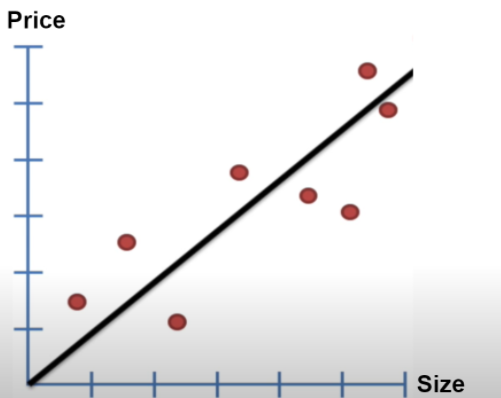So this is what we do in a linear regression algorithm. Then the question arises why do we need another regression algorithm like a locally weighted regression algorithm?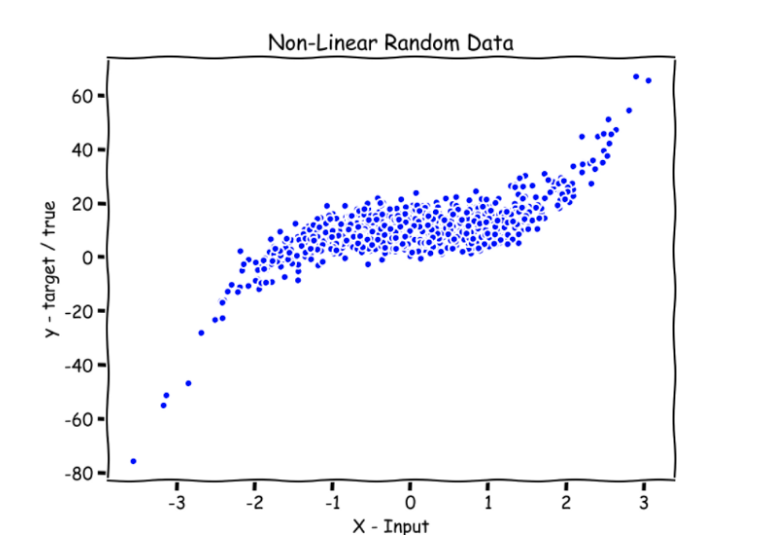Say we have a non-linear dataset, as shown in the image above. A standard line wouldn’t fit entirely into this type of dataset. In such a case, we have to introduce a locally weighted algorithm that can predict a very close value to the actual value of a given query point. So another thought coming to our mind is, let's break the given dataset into a smaller dataset and say we have multiple smaller lines that can fit the smaller dataset. Together, these multiple lines fit the entire dataset. To understand better, look at the diagram below.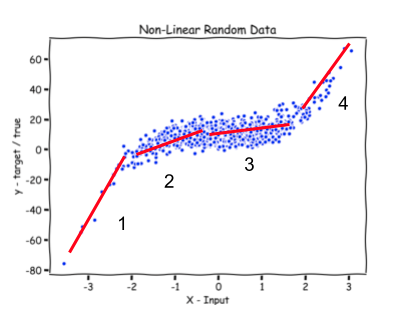Look at the 4 small lines that have been tried to fit smaller datasets and fit the entire dataset together. But now, the question arises of how to select the best line that can be chosen to predict the output for a given query point. Before we move on to find the best fit line, we must understand that we will always learn a different parameter for a particular query point. Hence locally weighted regression is a non-parametric algorithm.

NOTE: we will learn parameters in our algorithm for each point, and the above explanation is just for explanation purposes and to get an intuition of how we can think of building such a concept.

## Assigning weights to a line

We assume that there is a corresponding weight for that particular line given a query point. The one with the greatest weight will be the best fit line and will be used for predicting the output of a given query point. Now how to assign the weights is the next question? We know that if a given query point is close to a point on a particular line, it should have a greater weight than a far from the query point.  We define a weight function which is given as,x(i)  = any general training point,

x = given query point and,

𝝉 = is bandwidth parameter

The bandwidth parameter controls how quickly the weight should fall with the distance of the point with the training point. In simpler terms, it controls the width of how varied the data is. You can find the resemblance of the above function with the gaussian function.

Now assuming we fix the bandwidth parameter, we see that if we are given a query point close to a general training point, the weight would be close to 1 as the difference is close to 0. Similarly, if it’s too far from the training point, then the weight would be close to 0. Hence we choose this as our weight function.

## Mathematical Analysis

The above equation is the closed-form solution of locally weighted regression. Hence the value of the learned parameter or the solution of the equation is analogous to what we achieved in the linear regression.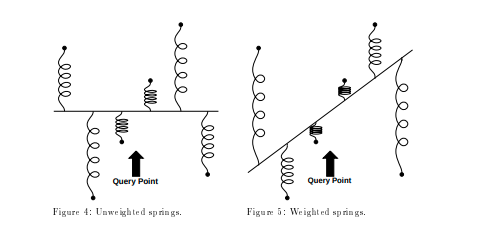Let’s look at the code of the Locally Weighted Regression.

## CODE IN PYTHON

Following is a self-implemented code of locally weighted regression and uses a small dataset downloaded from here.

import numpy as np # linear algebra
import pandas as pd # data processing
import matplotlib.pyplot as plt
import warnings
warnings.filterwarnings('ignore')
class LocallyWeightedRegression:
#maths behind Linear Regression:
# theta = inv(X.T*W*X)*(X.T*W*Y)this will be our theta whic will
# be learnt for each point
# initializer of LocallyWeighted Regression that stores tau as parameters
def __init__(self, tau = 0.01):
self.tau = tau
def kernel(self, query_point, X):
Weight_matrix = np.mat(np.eye(len(X)))
for idx in range(len(X)):
Weight_matrix[idx,idx] = np.exp(np.dot(X[idx]-query_point, (X[idx]-query_point).T)/(-2*self.tau*self.tau))
return Weight_matrix
# function that makes the predictions of the output of a given query point
def predict(self, X, Y, query_point):
q = np.mat([query_point, 1])
X = np.hstack((X, np.ones((len(X), 1))))
W = self.kernel(q, X)
theta = np.linalg.pinv(X.T*(W*X))*(X.T*(W*Y))
pred = np.dot(q, theta)
return pred
#function that fits and predicts the output of all query points
def fit_and_predict(self, X, Y):
Y_test, X_test = [], np.linspace(-np.max(X), np.max(X), len(X))
for x in X_test:
pred = self.predict(X, Y, x)
Y_test.append(pred)
Y_test = np.array(Y_test)
return Y_test
# function that computes the score rmse
def score(self, Y, Y_pred):
return np.sqrt(np.mean((Y-Y_pred)**2))
# function that fits as well as shows the scatter plot of all points
def fit_and_show(self, X, Y):
Y_test, X_test = [], np.linspace(-np.max(X), np.max(X), len(X))
for x in X_test:
pred = self.predict(X, Y, x)
Y_test.append(pred)
Y_test = np.array(Y_test)
plt.style.use('seaborn')
plt.title("The scatter plot for the value of tau = %.5f"% self.tau)
plt.scatter(X, Y, color = 'red')
plt.scatter(X_test, Y_test, color = 'green')
plt.show()
# reading the csv files of the given dataset
# store the values of dataframes in numpy arrays
X = dfx.values
Y = dfy.values
# normalising the data values
u = X.mean()
std = X.std()
X = ((X-u)/std)
tau = 0.2
model = LocallyWeightedRegression(tau)
Y_pred = model.fit_and_predict(X, Y)
model.fit_and_show(X, Y)

Output

### Effect of Tau/ Bandwidth Parameter

There has been a lot of literature written on this parameter, but we will discuss the essential points only. Let’s first plot the curves for different values of ‘tau.’

tau_values = [0.00001, 0.0001, 0.01, 0.01111, 0.1,0.2,  0.5, 1, 5, 10, 100]
for t in tau_values:
model = LocallyWeightedRegression(tau = t)
Y_pred = model.fit_and_predict(X, Y)
model.fit_and_show(X, Y)

(a)tau = 0.00001                                  (b) = 0.0001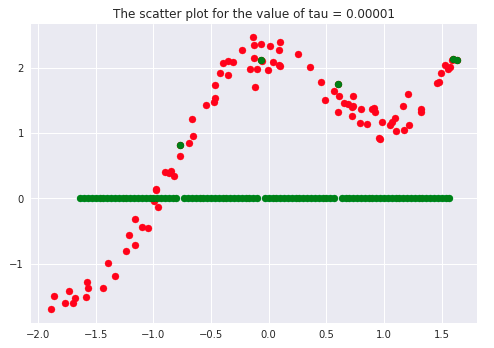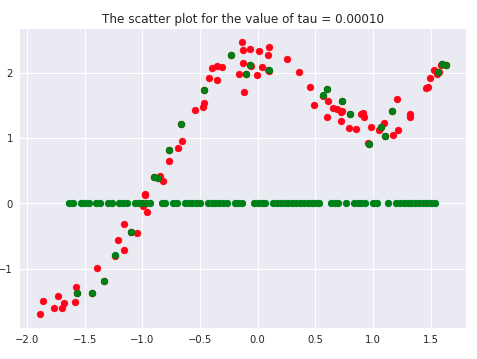(c) tau = 0.01111                               (d) tau = 0.1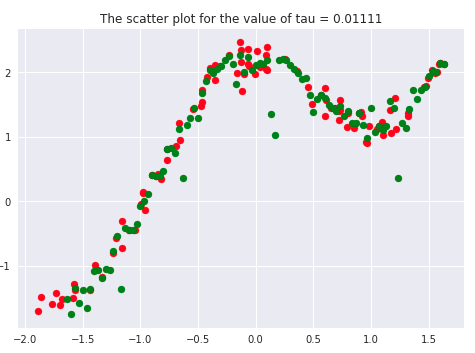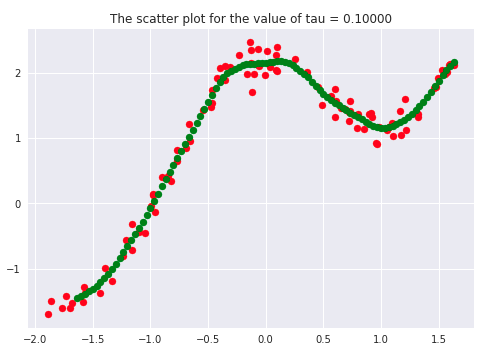(e) tau = 0.2                                       (f) tau = 0.5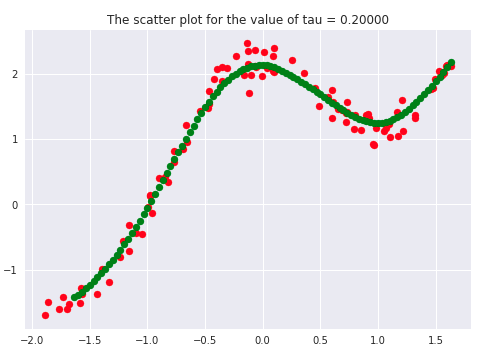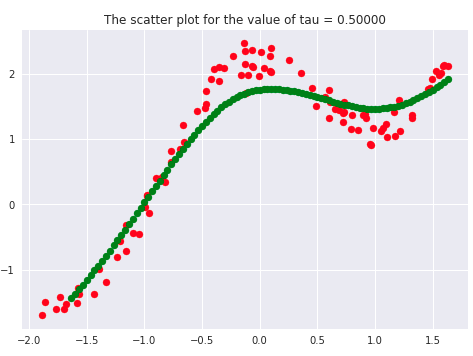(g) tau = 1.0                                       (h) tau = 5.0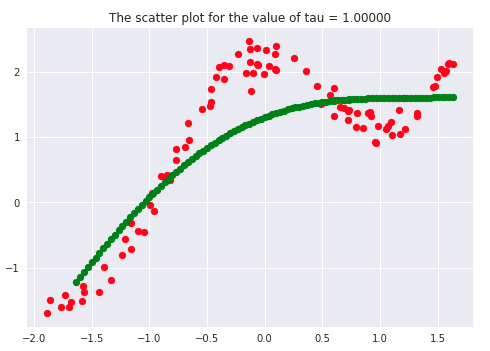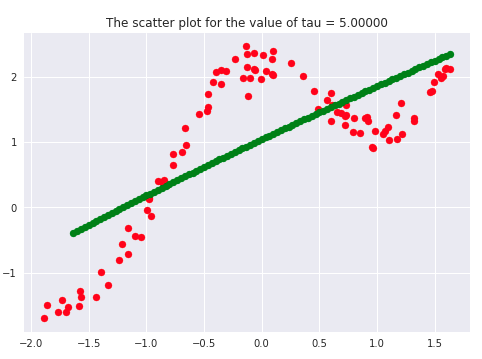(i) tau = 10.0                                   (j) tau = 100.0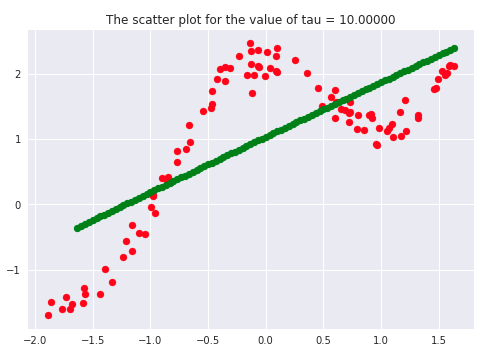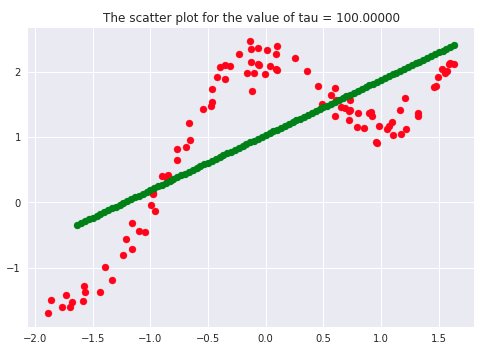If we carefully analyze the plots, we infer that the curve starts getting flatten as we increase. Also, the best fit line we get is for our dataset is when tau = 0.2. Another thing to observe is that smaller tau values fit the data well than larger tau values. Ultimately we’ll end up with straight lines if we keep increasing tau, as we can see from the plots.

Say  𝝉 >> 1, and say (x(i) - x)2  is fixed then, we can say that w(i) → 1 since the exponent term would be tending to 0. Hence the equation would just become the same as that used in simple linear regression. Therefore tau is controlling the width of the neighborhood in which the query point would lie. At the same time, it’s also responsible for underfitting or overfitting the data.

## When should we use Locally Weighted Regression?

Typically, think of linear regression initially as it is the most simple algorithm in all sense. But the issue arises if the linear regression is not fitting well enough or, say that the data follows a non-linear relationship, it will be underfitting and cannot be a reliable model for that case. Then we can switch on to locally weighted regression, which can give good results to some extent.

Typically this algorithm can be used when the dataset is dimensionally small, i.e., a small number of features, say 2 or 3. From experience, it has been seen that the locally weighted regression works relatively well for thousands of data points. Still, when we think of millions of data points, other sophisticated algorithms and data structures can handle these, for example, KD-trees.

## Advantages of Locally Weighted Regression

• It is a simple algorithm that works on the same idea of minimizing the least-squared error function.
• It can give excellent results when we have non-linear data points, and features are less, i.e., 2 or 3, and we want to incorporate all features in our analysis.
• Since it’s a non-parametric algorithm, there is no training phase.

## Disadvantages of Locally Weighted Regression

• Doesn’t work very well for high-dimensional data.
• Uses local fitting of data points at high computational costs.
• It’s very prone to the effect of outliers.

1. What are the kernel functions that we can use in Locally weighted Regression?
Ans. Typically, the gaussian kernel gives the best results, but we can use other kernel functions like the tri-cubic kernel.

2. What kind of algorithm is Locally weighted regression?
Ans. Locally weighted regression is a non-parametric algorithm that retains the training data at the time of predicting values.

3. What to use Locally weighted regression?
Ans. Locally weighted regression is typically used when we have small dimensional data and a few thousand data points.

## Kay takeaways

This article gave a brief explanation about the Locally Weighted Regression. We discussed intuition to Locally Weighted Regression, the mathematical analysis involved in Locally Weighted Regression is explained in detail. Apart from that, the self-implementation of Locally Weighted Regression is explained with proper analysis of the hyper-parameters. To dive deeper into machine learning, check out our industry-level courses on coding ninjas.

Happy Learning Ninja!